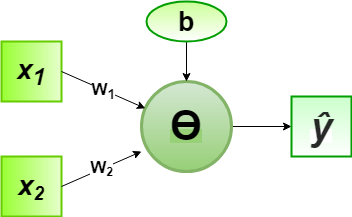# Implementation of Perceptron Algorithm for OR Logic Gate with 2-bit Binary Input

In the field of Machine Learning, the Perceptron is a Supervised Learning Algorithm for binary classifiers. The Perceptron Model implements the following function:For a particular choice of the weight vectorand bias parameter, the model predicts outputfor the corresponding input vector.

OR logical function truth table for 2-bit binary variables, i.e, the input vectorand the corresponding output0 0 0
0 1 1
1 0 1
1 1 1

Now for the corresponding weight vectorof the input vector, the associated Perceptron Function can be defined as:For the implementation, considered weight parameters areand the bias parameter is.

Python Implementation:

 # importing Python library  import numpy as np     # define Unit Step Function  def unitStep(v):      if v >= 0:          return 1     else:          return 0    # design Perceptron Model  def perceptronModel(x, w, b):      v = np.dot(w, x) + b      y = unitStep(v)      return y     # OR Logic Function  # w1 = 1, w2 = 1, b = -0.5  def OR_logicFunction(x):      w = np.array([1, 1])      b = -0.5     return perceptronModel(x, w, b)     # testing the Perceptron Model  test1 = np.array([0, 1])  test2 = np.array([1, 1])  test3 = np.array([0, 0])  test4 = np.array([1, 0])     print("OR({}, {}) = {}".format(0, 1, OR_logicFunction(test1)))  print("OR({}, {}) = {}".format(1, 1, OR_logicFunction(test2)))  print("OR({}, {}) = {}".format(0, 0, OR_logicFunction(test3)))  print("OR({}, {}) = {}".format(1, 0, OR_logicFunction(test4)))

Output:

OR(0, 1) = 1
OR(1, 1) = 1
OR(0, 0) = 0
OR(1, 0) = 1


Here, the model predicted output () for each of the test inputs are exactly matched with the OR logic gate conventional output () according to the truth table for 2-bit binary input.
Hence, it is verified that the perceptron algorithm for OR logic gate is correctly implemented.

Attention geek! Strengthen your foundations with the Python Programming Foundation Course and learn the basics.

To begin with, your interview preparations Enhance your Data Structures concepts with the Python DS Course.

My Personal Notes arrow_drop_upCheck out this Author's contributed articles.

If you like GeeksforGeeks and would like to contribute, you can also write an article using contribute.geeksforgeeks.org or mail your article to contribute@geeksforgeeks.org. See your article appearing on the GeeksforGeeks main page and help other Geeks.

Please Improve this article if you find anything incorrect by clicking on the "Improve Article" button below.